#### Mechanics, Strength of Materials and Theory of Structures

SHEAR FORCE & BENDING MOMENT
PURE BENDING
SOLVED EXAMPLES BASED ON SHEAR FORCE AND BENDING MOMENT DIAGRAMS
FLEXURAL STRESS

## SHEAR FORCE & BENDING MOMENT

SHEAR FORCE & BENDING MOMENT

16.1 Introduction

Shear force and bending moment diagrams are analytical tools used in conjunction with structural analysis in structural design, determining the value of shear force and bending moment at a given point of an element. Using these diagrams the type and size of a member of a given material can be determined.

16.2 Shear Force

Shear force at a point or at a given section is defined as the algebraic sum of vertical forces (Including the reactions) either to the left or right to the sections.

Shear force is different at different sections sections. To know the variation of shear force we draw shear force diagrams.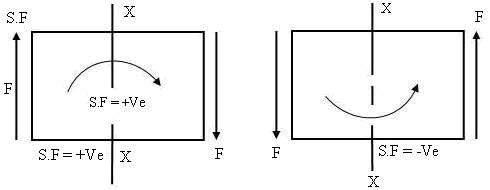Fig. 16.1 Shear force

Shear force may be considered to be +Ve if it is acting in the upward direction of the left hand side of the section (or) when it is acting in the downward direction on the right hand side (or) section & vice-versa.

16.3 Bending Moment

Bending moment at a section of beam is the algebraic sum of moment of forces to the right or left of the section. It Acts in a plane as axis (in axis). Bending moments either to the left (or) right of the section. Sign convention for shear force & bending moment.

Bending moment is said to be +ve when it is acting in the C.W (clock wise) as L.H.S (Left hand side) of the section (or) when it is acting in the A.C.W direction on the R.H.S as the section & vice versa.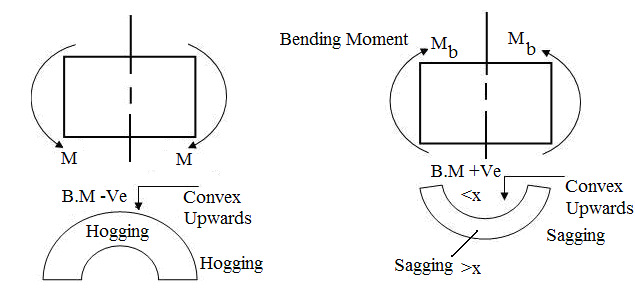Fig. 16.2 Bending moment

16.4 Shear Force Diagram and Bending Moment for Cantilever Beams

16.4.1 Cantilever beam with point load at the free end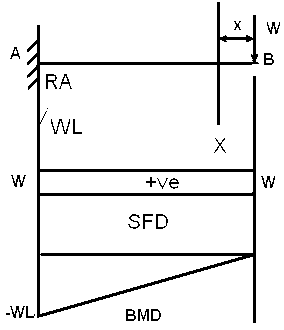Fig. 16.3 Cantilever beam

In cantilever beam we start from free end let us considered X-X section at a distance of x from free end.A.16.3_Cantilever_beam_point_load.swf

Reaction RA is given by the height S.F.D.

SHEAR FORCE at X-X,

S.FX-X=W

Moment at X-X,

MX-X=-WX

At B,

X=0,

(S.F)B=W

MB=0

At A,

X=L,

(S.F)A=W

MA=-WL

16.4.2 Cantilever beam is subjected to the moment at the free end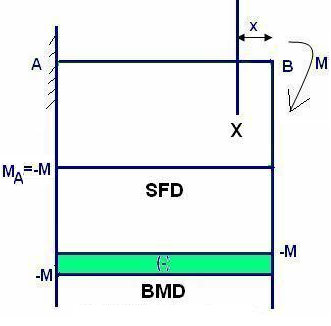Fig. 16.4 Cantilever beam

Mx=-M

MB=-M

(S.F)X-X=0

At B,

MB=-M

SFB=0, SFA=0

Whenever there is point load acting there is a sudden change in S.F.D

Similarly whenever a concentrated moment is acting there is a sudden change is S.F.D

In presence of point load there is a sudden change in S.F.D at that corresponding point.

In presence of concentrated moment there is a sudden change in B.M.D at that corresponding point.

16.4.3 Cantilever beam subjected to the moment at the middle point of the beam

MX=-M

MA=-M

MC=-M

MB=-MFig. 16.5 Middle point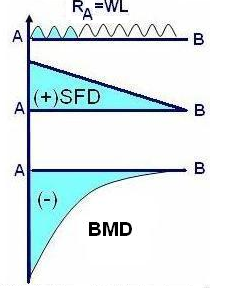At section X-X

SFX-X=WX

MX-X=-WX2/2

At A, X=L

SFA=WL

MA=-WL2/2

At B

SFB=0

MB=0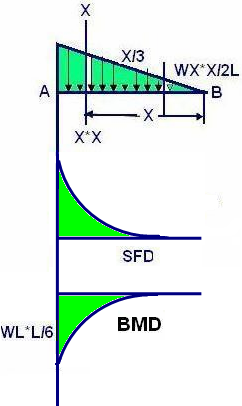B.M variation is always more than first order of S.F variation

Always cantilever beams we get first order type parabola and simply supported beam we get second order type parabola.

At X-X

(SF)X=WX2/2L

MX-X=-WX3/6L

At B, X=0

(SF)B=0

MB=0

At A, x=L

SFA=WL/2

MA=-WL2/6

16.5 S.F.D and B.M.D for Simply Supported Beams

16.5.1 Simply supported beam subjected to point load at mid section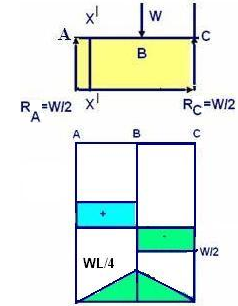Fig. 16.8 S.F.D and B.M.D

RA=W/2, RC=W/2 AB: (x: 0 to L/2)

(SF)X-X=+W/2

(BM)X-X=(W/2)X

SFA=W/2 BMA=0

SFB=W/2, MB=WL/4

BC (X: L/2-L)

(SF)X-X=W/2-W=-W/2

(BM)X-X=W/2(X)-W(X-L/2)

SFB=-W/2

MB=WL/4

SFC=-W/2

MC=WL/2-WL/2=0

16.5.2 Simply supported beam carrying uniform distributed loadA.16.9_Simply_supported_beam_carrying_UDL.swfFig. 16.9 Simply supported beam carrying UDL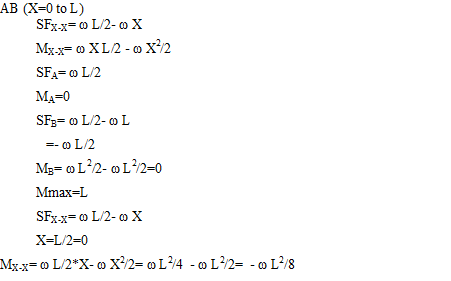16.5.3 Simply supported beam with point load at a distance ‘a’ from point A.A.16.10_Simply_supporterd_beam_with_point_load_at_A_distance.swf

Taking moments of forces at C of the beam the beam about A.Fig. 16.10 Simply supported beam with point load

Rb .l = Wa.

Rb = Wa/l

Ra = W-Wa/l

= W(l-a)/l

Ra = Wb/l { a+b = l}

For any section between A&C

Shear force

S.Fx = Ra

= Wb/l.

For any section b/w C&B

S.Fx = +Vb = + (Wa/l).

At any section between A&C distance x from

At any section b/w A&C distance x from

B.Mx = +(Wb /1) .x

{B.M = Bending moment}

X = 0 → Mx = 0

X = a → Mx = (Wab/l)

Hence bending moment increases uniformly from zero at the end A to Wab/l at C and B.M will decreases uniformly from Wab/l at C to zero at the end B.